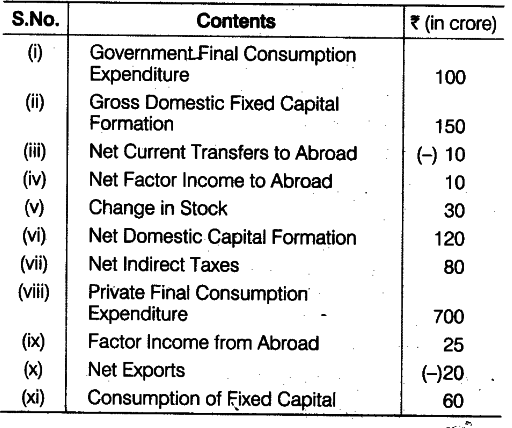# Calculate the National Income,

Calculate:
(a) National Income and (b) Gross National Disposable Income from the following data:(a) National Income (\$NN{{P}{FC}}\$)
= Private Final Consumption Expenditure + Government Final Consumption Expenditure + Net Domestic Capital Formation + Net Exports - Net Indirect Taxes - Net Factor Income to Abroad
= 700+100+120+(-20) - 80 -10 = 920-110 =Rs. 810 crore
(b) Gross National Disposable Income
= \$NN{{P}
{FC}}\$+ Consumption of Fixed Capital + Net Indirect Taxes - Net Current Transfers to Abroad =810 + 60 + 80 - (-10)
= Rs. 960 crore